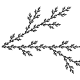Posted by: matheuscmss | January 31, 2012

## Zero Lyapunov exponents of the Hodge bundle

Giovanni Forni, Anton Zorich and I have just uploaded to ArXiv our paper “Zero Lyapunov exponents of the Hodge bundle”. Here, we accomplish the promise made in this post here (and in Appendix B of this paper here), and we exhibit an example of a square-tiled surface such that the Kontsevich-Zorich cocycle over its$PSL(2,\mathbb{R})$-orbit has a neutral Oseledets bundle despite the irreducibility of the cocycle. As we briefly explained in this previous post here, the existence of a non-trivial neutral Oseledets subbundle in this example follows from simple features of cocycles of matrices preserving pseudo-Hermitian forms of signature$(p,q)$, i.e., cocycles of$U(p,q)$ matrices, while the irreducibility of the cocycle follows by computing the Kontsevich-Zorich cocycle along certain pseudo-Anosov (periodic) orbits.

The article is organized as follows. In the first two sections we present the main example and state the main results, namely, existence of neutral Oseledets bundle, computation of Lyapunov spectrum of the example, and irreducibility of the cocycle. In the third section, we analyze the$PSL(2,\mathbb{R})$-orbit of the example to show that a formula of A. Eskin, M. Kontsevich and A. Zorich can be used to compute the sum of (non-negative) Lyapunov exponents by means of the so-called Siegel-Veech constants. Moreover, we claim that the knowledge of the sum of exponents in this case can be used to determine individual exponents due to the properties of cocycles of$U(p,q)$ matrices, and we postpone the proof of these properties to Appendix A of the article. Then, in the fourth section we quickly discuss some folklore facts about$PSL(2,\mathbb{R})$-orbits of square-tiled surfaces (implicitly used in SAGE programs by A. Zorich and V. Delecroix to numerically compute Lyapunov exponents), and we use this in the fifth section to reduce the irreducibility of the cocycle in our example to a statement about periodic orbits whose proof (based on a straightforward but somewhat long computation) is presented in Appendix B (who contains most of the figures of the article). Finally, we close the article by showing a class of examples (generalizing our initial one) where the sum of Lyapunov exponents can be computed by the method (of calculating Siegel-Veech constants) above.

Since most of the material of the article is either discussed previous in this blog and this research announcement here (e.g., the first two sections and Appendix A) or largely illustrated with figures (e.g., Appendix B) in the article itself, I will close this post here by saying that I plan to write a future post on how the “folklore” material of the fourth section of this article can be used to implement SAGE programs to numerically test Lyapunov exponents of the Kontsevich-Zorich cocycle associated to square-tiled surfaces.

## Responses

1.I am very interested in see numerical experiments illustrating these calculations. With square-tiled surfaces, you can lift the geodesic flow on the torus and keep track of which square you are on. Also to find the interval exchange map on a line transverse to the geodesic flow (a straight line basically).

This site uses Akismet to reduce spam. Learn how your comment data is processed.